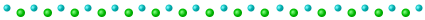# day 2

input

--- Day 2: Dive! ---

Now, you need to figure out how to pilot this thing.

It seems like the submarine can take a series of commands like `forward 1`, `down 2`, or `up 3`:

• `forward X` increases the horizontal position by X units.
• `down X` increases the depth by X units.
• `up X` decreases the depth by X units.

Note that since you're on a submarine, down and up affect your depth, and so they have the opposite result of what you might expect.

The submarine seems to already have a planned course (your puzzle input). You should probably figure out where it's going. For example:

``````forward 5
down 5
forward 8
up 3
down 8
forward 2
``````

Your horizontal position and depth both start at 0. The steps above would then modify them as follows:

• `forward 5` adds 5 to your horizontal position, a total of 5.
• `down 5` adds 5 to your depth, resulting in a value of 5.
• `forward 8` adds 8 to your horizontal position, a total of 13.
• `up 3` decreases your depth by 3, resulting in a value of 2.
• `down 8` adds 8 to your depth, resulting in a value of 10.
• `forward 2` adds 2 to your horizontal position, a total of 15.

After following these instructions, you would have a horizontal position of 15 and a depth of 10. (Multiplying these together produces 150.)

Calculate the horizontal position and depth you would have after following the planned course. What do you get if you multiply your final horizontal position by your final depth?

Result

1762050

--- Part Two ---

Based on your calculations, the planned course doesn't seem to make any sense. You find the submarine manual and discover that the process is actually slightly more complicated.

In addition to horizontal position and depth, you'll also need to track a third value, aim, which also starts at 0. The commands also mean something entirely different than you first thought:

• `down X` increases your aim by X units.
• `up X` decreases your aim by X units.
• `forward X` does two things:
• It increases your horizontal position by X units.

Again note that since you're on a submarine, down and up do the opposite of what you might expect: "down" means aiming in the positive direction.

Now, the above example does something different:

• `forward 5` adds 5 to your horizontal position, a total of 5. Because your aim is 0, your depth does not change.
• `down 5` adds 5 to your aim, resulting in a value of 5.
• `forward 8` adds 8 to your horizontal position, a total of 13. Because your aim is 5, your depth increases by 8*5=40.
• `up 3` decreases your aim by 3, resulting in a value of 2.
• `down 8` adds 8 to your aim, resulting in a value of 10.
• `forward 2` adds 2 to your horizontal position, a total of 15. Because your aim is 10, your depth increases by 2*10=20 to a total of 60.

After following these new instructions, you would have a horizontal position of 15 and a depth of 60. (Multiplying these produces 900.)

Using this new interpretation of the commands, calculate the horizontal position and depth you would have after following the planned course. What do you get if you multiply your final horizontal position by your final depth?

Result

1855892637